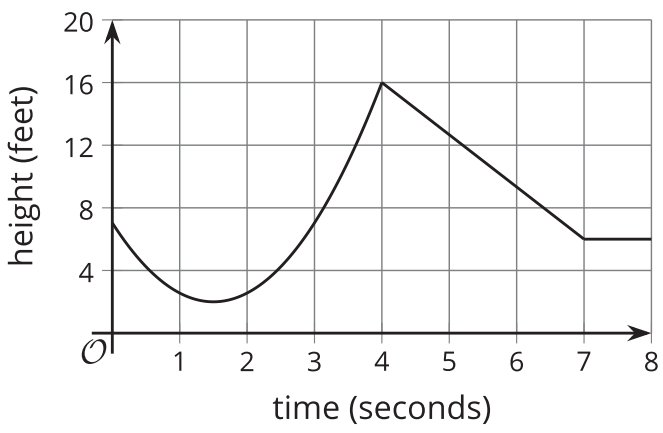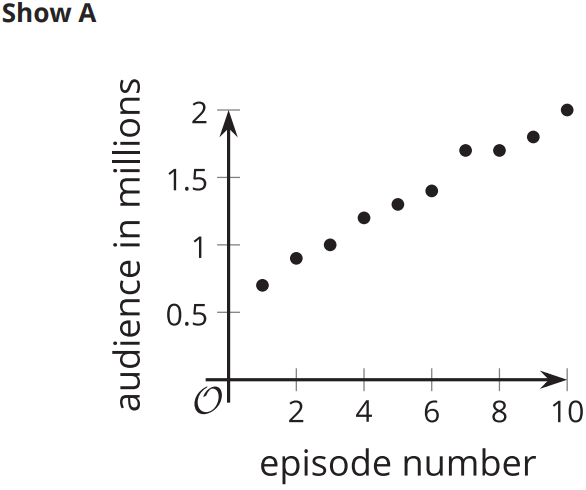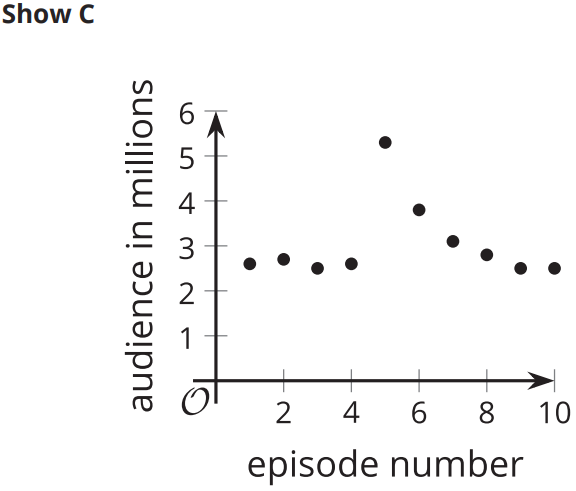# A.4.10 Practice Problems

## Problem 5

The graph of function f passes through the coordinate points (0,3) and (4,6). Use function notation to write the information each point gives us about function f.

## Problem 6

Two functions are defined by the equations f(x)=5-0.2x and g(x)=0.2(x+5). Select all statements that are true about the functions.

Check all that apply

## Problem 7

Match each feature of the graph with the corresponding coordinate point. If the feature does not exist, choose “none”.A. maximum B. minimum C. vertical intercept D. horizontal intercept 1. (0,7) 2. (1.5,2) 3. (4,16) 4. none

## Problem 8

The graphs show the audience, in millions, of two TV shows as a function of the episode number.For each show, pick two episode numbers between which the function has a negative average rate of change, if possible. Estimate the average rate of change, or explain why it is not possible.